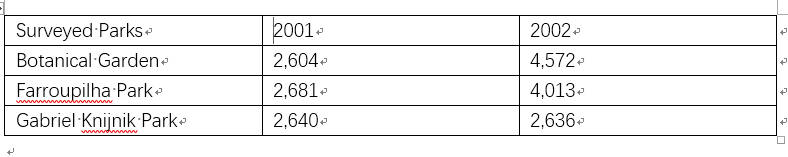SAT官方每日一题附答案和解析[数学](2019年8月23日)

(单词翻译:单击)

Mathematics>Standard Multiple Choice

A biologist conducts a study regarding an invasive species, the Gupta fly, in the city of Porto Alegre, Brazil. The flies were sampled from three different parks in 2001 and 2002.

Frequency of Sampled Gupta Flies in Porto Alegre by YearBased on the information in the table, as a percentage, approximately what is the probability that one of the sampled Gupta flies in 2001 was found in either the Botanical Garden or Farroupilha Park?

A.33%
B.50%
C.67%
D.77%

Choice C is correct. Using the information provided in the table, we find:

The total number of Gupta flies sampled in 2001 is 2604+2681+2640=7925.

The number of Gupta flies that were sampled in either the Botanical Garden or Farroupilha Park is 2604+2681=5285.

The probability that one of the sampled Gupta flies in 2001 was found in either the Botanical Garden or Farroupilha Park is the ratio of the number of Gupta flies sampled in these two parks over the total number of Gupta flies sampled in 2001. Calculate this ratio as a percentage:

Probability=5285/7925≈0.67=67%

Therefore, the probability that one of the sampled Gupta flies in 2001 was found in either the Botanical Garden or Farroupilha Park is approximately 67%.